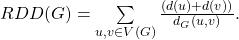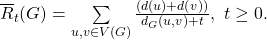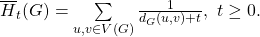# On the reformulated reciprocal degree distance of graphs

### Full PDFcreative_2016_25_2_205_213

The reciprocal degree distance (RDD), defined for a connected graphas vertex-degree-weighted sum of the reciprocal distances, that is,The new graph invariant named reformulated reciprocal degree distance is defined for a connected graphasThe reformulated reciprocal degree distance is a weight version of the-Harary index, that is,In this paper, the reformulated reciprocal degree distance and reciprocal degree distance of disjunction, symmetric difference, Cartesian product of two graphs are obtained. Finally, we obtain the reformulated reciprocal degree distance and reciprocal degree distance of double a graph.